# 7). Vertical equipotential lines are equallyspaced 2 cm apart. At the zero volt line,-10VoV10V20Vcalculate the magnitude and direction ofthe electric field.

Question
18 views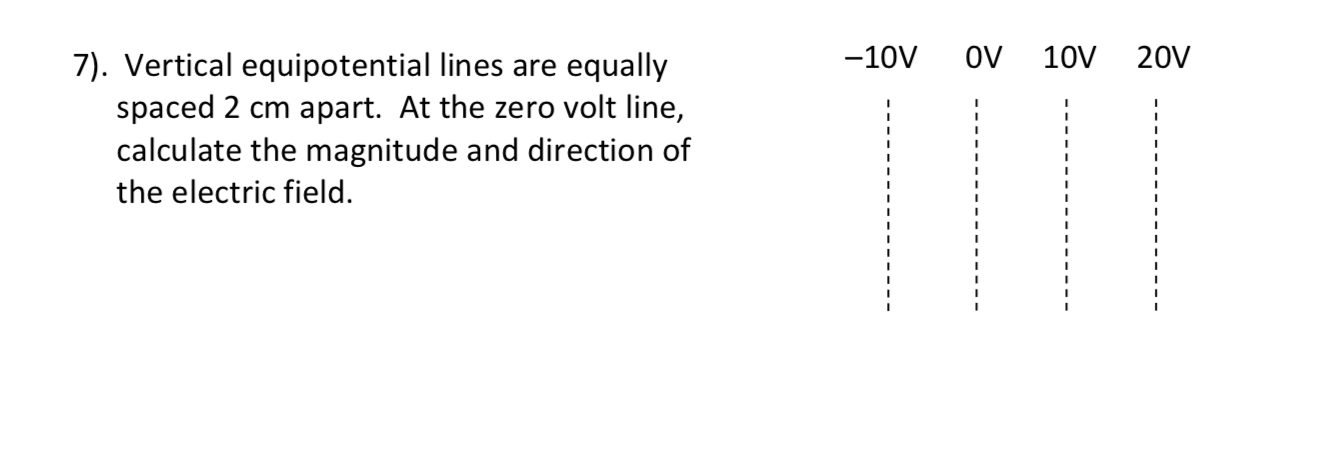help_outlineImage Transcriptionclose7). Vertical equipotential lines are equally spaced 2 cm apart. At the zero volt line, -10V oV 10V 20V calculate the magnitude and direction of the electric field. fullscreen
check_circle

star
star
star
star
star
1 Rating
Step 1

Electric field is a measure  of  electric force per unit charge.

The Electric field direction is taken to be the direction of the force it would exert on a positive test charge.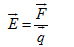It is a vector quantity.

Electrical Potential at a point in an electric field is equal to the amount of work to be done to bring a unit positive electric charge from infinity to that point.

Generally, it is denoted by V.

It is a scaler quantity.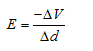Where,

∆V= Change in voltage

∆d= distance between the two electric lines.

Equipotential Surface refers to a region where every point in it is at the same potential.

The direction of electric field is always perpendicular to the equipotential surface.

It is given that the space between the each equipotential surface is 2 cm.

In the given question, we have to find the magnitude and direction of electric field at zero voltage line.

Step 2

First we have to find the direction of the electic field at zero potential line.

The direction of the electric field is always from higher to lower potential as shown: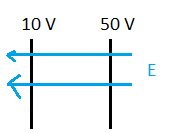Using the above concept for our question,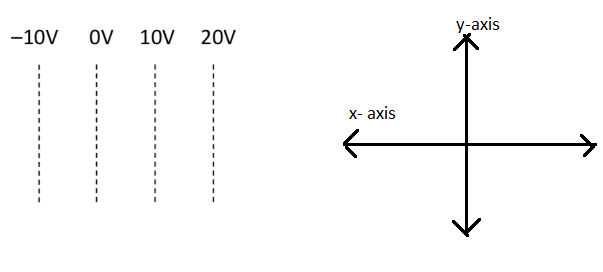The electric field direction due to 10 V at 0 V line is towards – x axis.

The electric field direction due to 20 V at 0 V line is towards – x axis.

The electric field direction due to -10 V at 0 V line is towards – x axis.

Hence, the net electric field direction at 0 V line is towards – x axis( or in left direction).

Step 3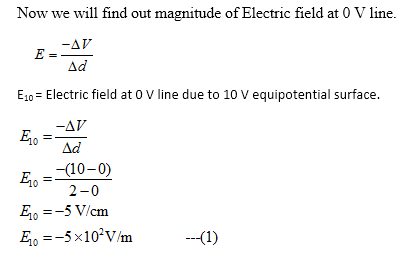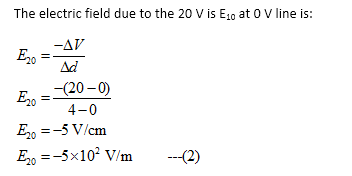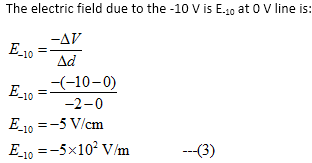...

### Want to see the full answer?

See Solution

#### Want to see this answer and more?

Solutions are written by subject experts who are available 24/7. Questions are typically answered within 1 hour.*

See Solution
*Response times may vary by subject and question.
Tagged in